International Journal of Applied Mathematics and Theoretical Physics
Volume 2, Issue 4, October 2016, Pages: 52-56

Classical Derivation of the Total Solar Deflection of Light

Steven D. Deines

Donatech Corporation, Inc., Fairfield, Iowa, USA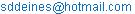Steven D. Deines. Classical Derivation of the Total Solar Deflection of Light. International Journal of Applied Mathematics and Theoretical Physics. Vol. 2, No. 4, 2016, pp. 52-56. doi: 10.11648/j.ijamtp.20160204.15

Received: August 7, 2016; Accepted: September 23, 2016; Published: October 19, 2016

Abstract: It has been held that the total solar deflection of light could only be derived correctly by Einstein’s general theory of relativity. This paper provides a new classical derivation for the total gravitational deflection of light as a photon passes by the Sun. Newton, Cavendish, Einstein and others discussed or calculated how gravity may bend the paths of light. In particular, von Soldner published an incomplete classical derivation to predict a solar deflection that was half of the later observed value, since he assumed a light wave was deflected by a stationary Sun. Einstein’s earliest derivation used his equivalence principle of a homogeneous gravity field and a constant dynamical acceleration, which predicted half of the observed solar deflection angle, because he was then unaware of all the first-order space-time contributions. Einstein’s general relativity theory predicted the full solar deflection. Assuming the photon has a mass via Einstein’s mass-energy equation, this classical derivation uses Newton’s mechanical laws and his law of gravitation for the photon’s and the Sun’s hyperbolic paths about their mutual barycenter. Both the Sun and photon deflect each other about their barycenter with an infinite lever. This Newtonian derivation obtains the prediction of 1.75² against the celestial sphere for the full gravitational deflection of light relative to the Sun.

Keywords: Photon Deflection, Solar Deflection, General Relativity

1. Introduction

Several scientists discussed or derived the possible deflection of light by gravity. Newton  was one of the first to expect a gravitational bending of the paths of light corpuscles in his Principia and later in his description of bending light in his Opticks. Mitchell  studied gravitational horizons and the spectral shift of light due to gravity. C. M. Will  describes how Henry Cavendish did his light bending calculations and gave details on von Soldner’s derivation  for the gravitational deflection of light passing the Sun. The critical flaw in von Soldner’s derivation was he assumed the Sun was stationary while a light wave from a star grazed by the Sun. Einstein [5, p. 454] argued that a region in free fall is really an inertial environment and that the rules of special relativity apply to a freely falling observer. This was the basis for his initial concept of equivalence between gravitational and inertial masses. Later, Einstein  calculated how a photon from a distant star with m = E/c2 could be deflected by the Sun’s gravity, but that calculation was only half of the correct value. He incorrectly assumed the rate of fall of a body in gravity alone would have the correction of Φ/c2 where Φ is the Sun’s gravitational potential, but the first order calculation should be 2Φ/c2 if the space components are accounted besides the time component. (The reader can read Nelson  for deriving the post-Newtonian approximation for an accelerated, rotating reference frame from Einstein’s general relativity to obtain the space-time metric equations that include the correct first order term of 2Φ/c2.) Einstein conceived that a closed box containing local experiments cannot distinguish between a homogeneous gravity field pulling on the stationary box in one direction and a constant translational acceleration pulling the box in the opposite direction without gravity. Eventually, Einstein  published his prediction for the Mercury perihelion effect from his general relativity theory with his additional prediction for the solar gravitational deflection of light. Any multiples of von Soldner’s predictions could have been possible—say three, p/2, but why 2 exactly? Will  claimed that classical derivations or predictions using the equivalence principle would get only half of the observed value, but that curved space-time is necessary to derive the remaining half of the deflection. Einstein wanted to derive the field equations for a general accelerated reference frame without having to use a preferred inertial frame. Einstein  already proposed that light energy was found in discrete quanta in the photoelectric effect. Later, he derived  the equivalence formula between mass and energy. Einstein recognized that the deflection would be nonzero and encouraged the astronomical community to measure the deflection as early as June 1911 [12, p. 183]. Eventually, two expeditions in 1919 confirmed the deflection of light in the neighborhood of the Sun to be at Sobral 1.98"±0.12" and Principe Island 1.61"±0.30’, which instantly made Einstein then the most famous physicist [12, p. 187]. The next section contains the derivation using Newton’s gravitational law to obtain the photon’s hyperbolic orbit relative to the barycenter.

2. Classical Derivation of the Photon’s Deflection Angle

Theoretically, a quantum of light has zero rest mass if it is stationary, because it has no effective energy to transport. If discrete quanta of energy obeyed Einstein’s equation, E = m c2, then a moving photon has an associated mass of m = E/c2 = hν/c2 where h is Plank’s constant, c is the speed of light in a vacuum, and n is the frequency of the photon. So, the photon from a distant star that is glancing by the Sun would have both a gravitational and a centripetal force as required by Newton’s third law, which the Sun’s gravity can now deflect the photon’s trajectory as it grazes by the Sun’s surface. The Sun and a single photon of an arbitrary frequency are assumed to have a fixed mass to four significant figures. For the deflection angle calculations to be completed to three significant figures, the astronomical constants have already been determined to much higher significance . The general solution to the two-body problem allows that the mass distributions of two orbiting bodies can be treated equivalently as point masses in the analysis. This ensures that neither body touches the other. The solution to the two-body problem incorporating Newtonian gravity has no classical limit on initial high velocity for either body placed in hyperbolic orbits.

The two body problem applies to the photon’s motion as it just passes the Sun traveling to the Earth observer. The basic differential equation of motion for Newton’s gravitational law is: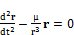(1)

where the reduced mass is μ = G(M + m) with G being the gravitational constant, M the Sun’s mass and m being the photon’s mass, while r is the vector to the photon from the mass center of both bodies, hereafter called the barycenter. Take the vector cross product of Equation (1) with r, reduce terms like r x r = 0, and obtain the derivative for a cross product, which equals zero. This leads to the definition of angular momentum h per unit mass, which is perpendicular to r and its derivative or velocity v.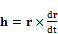with the magnitude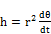(2)

Use Equation(1) to get dh/dt = 0, which shows h is conserved and normal to the plane of motion. Take the cross product between Equation (1) and h, apply the triple vector identity, and integrate the result directly to get: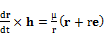(3)

where e is called the eccentricity vector and is a constant of integration. Take the dot product between Equation (3) and e to get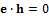, which places e in the orbital plane. To solve for Equation (1), take the dot product of Equation (3) with r, apply the triple scalar product identity, and obtain: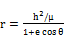where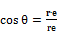(4)

This is the general form for a conic section with the origin at a focus. The vector e is parallel to the direction of minimum r. Follow the derivation of Kaplan  for the hyperbolic diagram in Figure 1.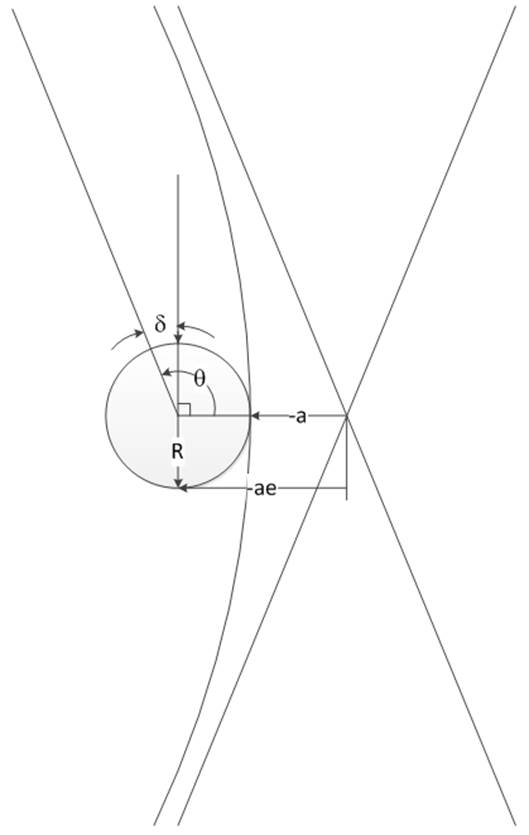Figure 1. Definition of Hyperbolic Parameters.

For the hyperbola, h2/μ = -a(e2-1) for negative semimajor axis, a, and the minimum r = R = -a(e-1), which also equals the semilatus rectum, from Figure 1. The derivative of Equation (4) with respect to r only obtains the radial velocity, which differs from the total velocity that has an additional transverse component.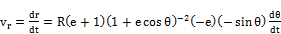(5)

Replace the theta derivative using Equation (2) and reduce to get: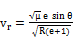(6)

Square Equation (6) and take the limit as r to get the total velocity at the asymptote. At infinity, the Sun has no gravity to affect the incoming photon’s velocity or coordinate time, which allows the substitution of v2 = c2 for light’s speed in a vacuum. From Equation (4) as r, then (1+e cos q)0, or cos θ = -1/e.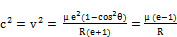(7)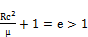or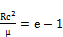(8)

In Figure 1 with a small angle δ,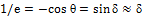. There is a deflection of δ for the incoming photon, and there is another deflection of δ after the photon passes the barycenter along the hyperbolic orbit approaching the other asymptote. The photon’s classical deflection is a total of 2δ with the result: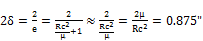(9)

This is the same as the final answer of von Soldner  and Einstein’s first derivation , but this is only half of the total classical derivation. Einstein’s equivalence principle is not applied, because a homogeneous gravity is not appropriate, and neither is the approximation by a freely falling frame.

3. Classical Derivation of the Sun’s Deflection Angle Relative to the Barycenter

The general solution of the two-body problem considers two physical masses, which are reduced to equivalent point masses in some inertial reference frame having X, Y and Z axes. The mass m1 is located at vector r1 and mass m2 is at r2. Let r = r1r2. The center of mass is found at rc by: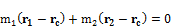(10)

Substitution for r1 and r2 will obtain the vector displacement from the center of mass to m1 and m2.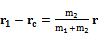, and                     (11)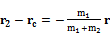(12)

Let the gravitational force from m1 be F1 and from m2 be F2. Then,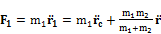, and              (13)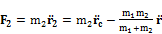(14)

F1 and F2 attract each other, and Newton’s third law requires F1 = -F2, which obtains the condition that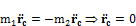(15)

The important point of Equation (15) is the center of mass of the two-body problem never accelerates. Newton’s first law allows this barycenter to have an initial velocity. Simply choose another inertial frame attached to this barycenter so that it is stationary (no net velocity) with no other external forces affecting the two-body problem. The barycenter, which is at the focus point of the two hyperbolic orbits, will be usually outside of the Sun, but when the Sun is near the periapsis of its hyperbolic orbit, the barycenter will be inside the Sun as illustrated in Figure 2.

The original problem is to derive the deflection of the photon relative to the Sun while the photon just grazes by the Sun. The photon begins at r = +∞ and ends at r = -∞ in this inertial frame, while the Sun begins at r = -∞ and ends at r = +∞ with the restriction that the barycenter remains stationary. Relative to the barycenter, the photon’s orbital periapsis is the radius of the spherical Sun, R. The deflection of the photon relative to the barycenter has been calculated with the result of 0.875". The other half of the problem is calculating the Sun’s deflection in its hyperbolic orbit relative to the barycenter.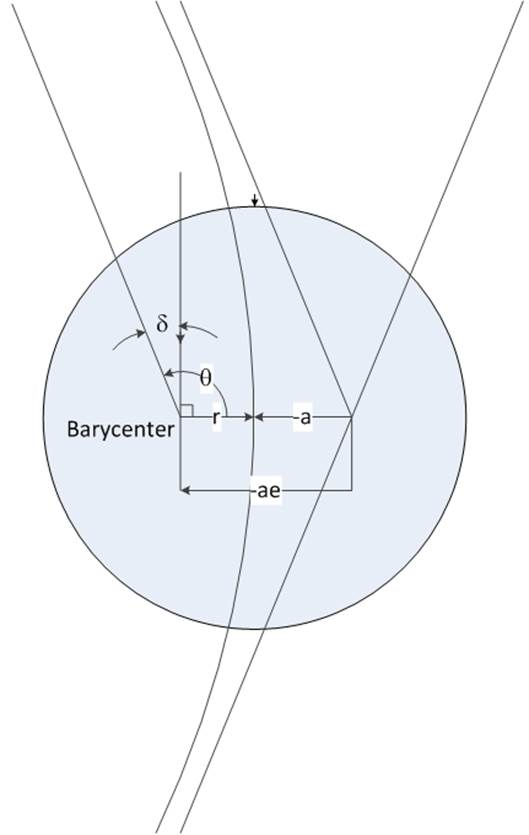Figure 2. Sun’s Hyperbolic Orbit by Barycenter (not scaled).

The position and velocity of a hyperbolic orbit has been solved [14, p. 302-304]. The equation for the Sun’s hyperbolic orbit is: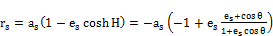(16)

At the periapsis, θ = 0, which obtains rs = (-as)(es-1) as the minimum distance, which is also the form for the photon’s minimum orbital distance [i.e. rp = R = (-ap)(ep-1)]. Equation (10) requires that the mass points and the barycenter be collinear. Using magnitudes, the balance equation mp rp = ms rs is obtained from Equation (10). In the limit as rs, then (1+es cos θ)0, or cos θ = -1/es. Equation (10) requires that in this limit, both the photon and Sun must be on the same asymptote line at an angle θ, which implies es = ep. The balance equation then obtains the ratio of as = (mp/ms) ap. So, the Sun’s hyperbolic orbit in terms of the semilatus rectum [i.e. p = (-a)(e-1)] is scaled by the factor mp/ms compared to the photon’s hyperbolic orbit, but both orbits have the same eccentricity e. Using the same mathematics for calculating the photon’s deflection compared to the barycenter, the Sun’s deflection will be the 0.875", the same as the photon’s, but with the opposite angular displacement. The two angles cause the total deflection of 1.75" of the photon relative to the Sun. A one-to-one onto correspondence exists between as and ap, so as approaches the same infinity as ap. Since the distances of the star and the Sun approach the same infinity relative to their mutual barycenter, the total angular displacement can be compared to a long teeter-totter in Figure 3. If the photon from the distant star was displaced by an angle θ, the vertical displacement along the left would be one unit, as indicated by one double arrow, relative to the fulcrum. If the Sun were on the right end of the teeter-totter, the Sun would be displaced downwards by the same angle θ that the photon was displaced upwards by θ. However, the star’s apparent vertical displacement is two units as indicated by the two double arrows relative to the horizontal dashed line at the Sun’s location. From the fulcrum’s point of view, the photon travels from - to + and is deflected up by θ, while the Sun is moved from + to - and is deflected down by θ, giving a total of 2θ for the final displacement. Thus, the photon’s total displacement against the celestial sphere relative to the Sun is 1.75" due to gravity. The two-body problem is relative to the barycenter, so one must compute the deflections of the photon and the Sun, each relative to the barycenter, to get the photon’s complete deflection relative to the Sun against the celestial sphere.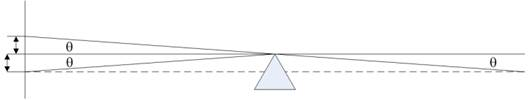Figure 3. Total Displacement of Photon and Sun Relative to Barycenter.

Shapiro et al  reported solar deflections of radio sources with a result of γ = 0.9998±0.0004 where γ is unity in general relativity, which predicts a ray grazing the Sun’s limb has a deflection of θ 1.75" [16, p. 1222]. The parameter g compares various theories in a post-Newtonian formalism. The team used Very-Long-Baseline Interferometry (VLBI) for very accurate observations of solar deflections. This classical derivation compares identically with the general relativity prediction for the total solar deflection.

4. Conclusion

Many physicists, including Newton, Cavendish, and Einstein, expected a photon to experience a deflection in its path going past a gravitational body on its way toward the Earth. For example, von Soldner published a derivation for a light wave deflected gravitationally by the stationary Sun that was half of later observed angular deflections caused by the Sun . Einstein used his equivalence principle in his calculations . He originally obtained half of the actual deflection, since he assumed the first order effect from the gravitational potential was Φ/c2 instead of 2Φ/c2, because he did not include the all the space-time contributions. His general relativity theory obtained the correct solar deflection of light passing by the Sun .

This paper obtains a Newtonian derivation by assuming the photon as a tiny mass will travel by the Sun in a hyperbolic orbit by passing the Sun at a distance of R, which is equal to the radius of the spherical Sun. However, this only gets half of the expected deflection between the photon and Sun, because the photon’s deflection is relative to the barycenter. The Sun also executes a hyperbolic orbit relative to the barycenter that temporarily encompasses the barycenter, because its periapsis is much closer to the barycenter as required by the balance equation. It is shown that the Sun’s hyperbolic orbit has the same eccentricity as the photon’s orbit, so the Sun’s deflection about the barycenter is also the same, being 0.875". Relative to the Sun, the photon’s effective deflection is a total of 1.75" against the celestial sphere, which is the same as measured by VLBI for the most accurate measurements using radio sources. This also answers the question why previous classical derivations were half of the observed deflection against the celestial sphere, because those derivations ignored the solar displacement as the Sun goes by the barycenter. Archimedes claimed he could move the world if given a place to stand a fulcrum and a long rigid lever . Apparently, no one before expected the photon would also deflect the Sun gravitationally with an infinite lever through the barycenter, which results in a maximum deflection of 1.75" against the celestial sphere of the photon relative to the Sun.

References

1. Newton, I., Philosophiae Naturalis Principia Mathematica, (1726), English Translation by Alexandre Koyr (1833) with variant readings as assembled and edited by Alexandre Koyr, Vol. 1, Harvard University Press, Cambridge Mass.
2. Michell, J. "On the means of discovering the distance, magnitude of the fixed stars ...", Philosophical Transactions of the Royal Society, p. 35-57, and Tab III (1784).
3. Will, C.M. "Henry Cavendish, Johann von Soldner, and the deflection of light", Am. J. Phys., 56, p. 413–415, (1988).
4. von Soldner, J. G. (1801), English translation "Johann Georg von Soldner and the gravitational bending of light" in Foundations of Physics, 8, p. 927-950, (1978).
5. Einstein, A. "Relativitätsprinzip und die aus demselben gezogenen Folgerungen (On the Relativity Principle and the Conclusions Drawn from It)", Jahrbuch der Radioaktivität (Yearbook of Radioactivity), 4: pp. 411–462. See page 454 (1908).
6. Einstein, A. "The effect of gravity on light" (1911), translated and reprinted in The Principle of Relativity, by Davis, F. A., Dover Publications (1928).
7. Nelson, R. A. "Post-Newtonian approximation for an accelerated, rotating frame of reference", General Relativity and Gravitation, 22, Issue 4, p. 431-449 (1990).
8. Einstein, A. "Explanation of the Perihelion Motion of Mercury from the General Theory of Relativity",Preussische Akademie der Wissenschaften, Sitzungsberichte, (part 2), p. 831–839(1915).
9. Will, C. M. "The Confrontation of General Relativity and Experiment", Living Reviews of Relativity, 9 (3), p. 39, (2006).
10. Einstein, A. "Über einen die Erzeugung und Verwandlung des Lichtes betreffenden heuristischen Gesichtspunkt(trans. On aHeuristic Viewpoint Concerning the Production and Transformation of Light)", Annalen der Physik, 17 (6), p. 132–148 (1905).
11. Einstein, A. "Ist die Trägheit eines Körpers von seinem Energieinhalt abhängig? (trans. Does the Inertia of a Body Depend Upon Its Energy Content?)", Annalen der Physik, 18 (13), p. 639–641 (1905).
12. Renn, J., [Ed.] Albert Einstein-Chief Engineer of the Universe: One Hundred Authors for Einstein, Wiley-VCH, Berlin, p. 178-187 (2005).
13. Seidelmann, P. K. [Ed.] Explanatory Supplement to the Astronomical Almanac, U.S. Naval Observatory, University Science Books (1992).
14. Kaplan, M. H. Modern Spacecraft Dynamics and Control, John Wiley & Sons, New York, p. 300-304 (1976).
15. Shapiro, S. S., Davis, J. L., Lebach, D. E., and Gregory, J. S. "Measurement of the Solar Gravitational Deflection of Radio Waves using Geodetic Very-Long-Baseline Interferometry Data, 1979-1999", Physical Review Letters, 92, No. 12, p. 121101-1 to 4 (2004).
16. Serway, R. A. and Jewett Jr., J. W., Physics for Scientists and Engineers, Cengage Learning, 9th ed. (2014).
17. Walton, F. R. "The Library of History of Diodorus Siculus", pub. in Loeb Classical Library, Vol. XI (1957).

 Contents 1. 2. 3. 4.
Article ToolsAbstractPDF(261K)